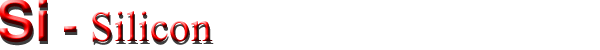Electrical properties

Basic Properties
Mobility and Hall Effect
Transport Properties in High Electric Field
Impact Ionization
Recombination Parameters
Surface Recombination

Basic Properties

 Breakdown field ≈3·105V/cm Mobility electrons ≤1400 cm2 V-1s-1 Mobility holes ≤450 cm2 V-1s-1 Diffusion coefficient electrons ≤36 cm2/s Diffusion coefficient holes ≤12 cm2/s Electron thermal velocity 2.3·105m/s Hole thermal velocity 1.65·105m/s

Mobility and Hall Effect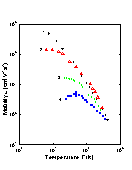Electron mobility versus temperature for different doping levels. 1. High purity Si (Nd< 10-12 cm-3); time-of-flight technique (Canali et al. ) 2. High purity Si (Nd< 4·10-13 cm-3): photo-Hall effect (Norton et al. ) 3. Nd= 1.75·1016 cm-3; Na = 1.48·1015 cm-3; Hall effect (Morin and Maita ). 4. Nd= 1.3·1017 cm-3; Na = 2.2·1015 cm-3; Hall effect (Morin and Maita ).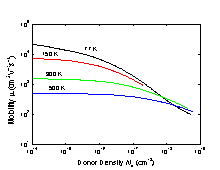Electron drift mobility versus donor density at different temperatures (Li and Thumber ).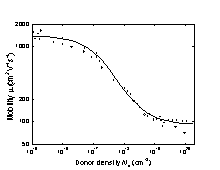Electron drift mobility versus donor density, T=300 K. (Jacoboni et al. ).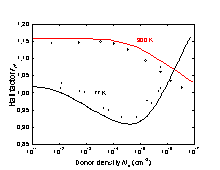The electron Hall factor versus donor density. 77 and 300 K. Solid lines show the results of calculations. Symbols represent experimental data (Kirnas et al. ).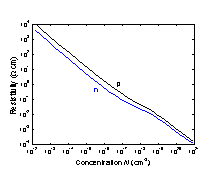Resistivity versus impurity concentration for Si at 300 K.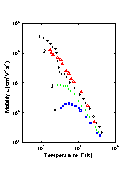Temperature dependences of hole mobility for different doping levels. 1. High purity Si (Na = 1012 cm-3); time-of-flight technique (Ottaviany et al. ); 2. High purity Si (Na~1014 cm-3); Hall-effect (Logan and Peters ) 3. Na=2.4·1016 cm-3; Nd=2.3·1015 cm-3; Hall-effect (Morin and Maita ) 4. Na=2·1017 cm-3; Nd=4.9·1015 cm-3; Hall-effect (Morin and Maita )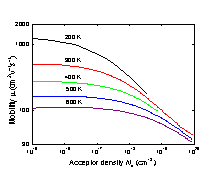Hole drift mobility versus acceptor density at different temperatures (Dorkel and Leturcq ).Hole drift mobility versus acceptor density. 300 K. (Jacoboni et al. ).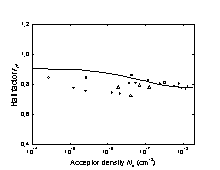The hole Hall factor versus acceptor density. 300 K. (Lin et al. ).

Transport Properties in High Electric Field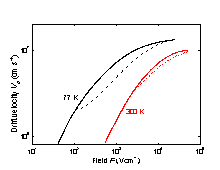Si. Electron drift velocity vs. electric field. Solid lines: F||(111). Dashed lines: F||(100). Jacoboni et al. (1977).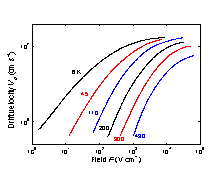Si. Electron drift velocity vs. electric field at different temperatures. F||(111). Jacoboni et al. (1977).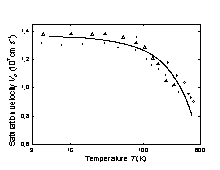Temperature dependence of the saturation electron drift velocity (Jacoboni et al. ). Solid line is calculated according to equation: vs=vso·[1+C·exp(T/Ι)]-1, where vso=2.4·107 cm s-1, C=0.8, Ι=600K.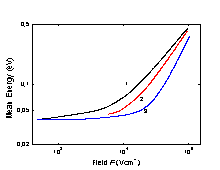Mean energy of electrons as a function of electronic field F at different donor densities. F||(111). 300 K. 1. Nd = 0; 2. Nd = 4·1018 cm-3; 3. Nd = 4·1019 cm-3. (Jacoboni et al. ).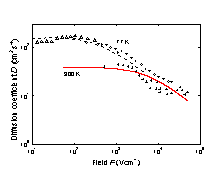The field dependence of longitudinal electron diffusion coefficient D for 77K and 300 K. F || (111). Dotted and solid lines show the results of Monte-Carlo simulation. Symbols represent measured data. (Canali et al. ).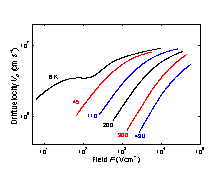Field dependences of the hole drift velocity at different temperatures. F || (100). (Jacoboni et al. ).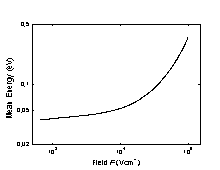Mean energy of holes as a function of electronic field F. Na = 0, T=300 K. (Jacoboni et al. ).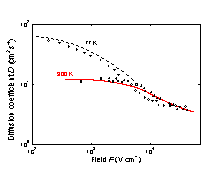The field dependence of longitudinal hole diffusion coefficient D for 77K and 300 K. F||(111). Dotted and solid lines show the results of Monte-Carlo simulation. Symbols represent measured data. (Canali et al. ).

Impact Ionization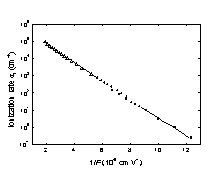Electron ionization rate αi vs. 1/F. T = 300 K. (Maes et al. ).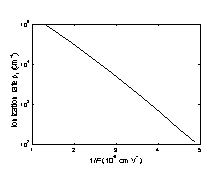Hole ionization rate βi vs. 1/F. T = 300 K. (Grant ).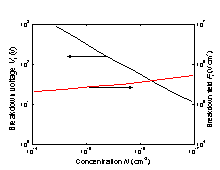Breakdown voltage and breakdown field vs. doping density for an abrupt p-n junction. T = 300 K. (Sze ).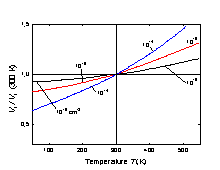Normalized breakdown voltage vs. temperature for an abrupt p-n junction at different doping levels. (Crowell and Sze ).

Recombination Parameters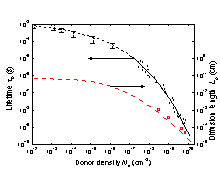Lifetime τp and diffusion length Lp of holes in n-type Si vs. donor density. T = 300 K. For 1012 cm-3 < Nd ≤ 1017 cm-3- from numerous experimental data for good quality industrial produced n-Si. For Nd ≥ 1017 cm-3 - (Alamo and Swanson ). Lp (Na) dependence (dashed line) is calculated as Lp(Nd)=[Dp(N)·τp(N)]1/2, where Dp=(kB·T/q)·μp.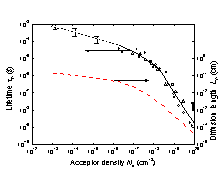Lifetime τn and diffusion length Ln of electrons in p-type Si vs. acceptor density. T = 300 K. For 1013 cm-3 < Na≤1016 cm-3 - from numerous experimental data for good quality industrial produced p-Si. For Na ≥ 1016 cm-3 - (Tyagi and Van Overstraeten ). Ln(Na) dependence (dashed line) is calculated as Ln(Na)=[Dn(N)·τn(N)]1/2, where Dn=(kb·T/q)·μn.

Surface recombination

Surface recombination rate depending on treatment of Si surface lies in the range between 102 ÷(6-8)·104cm/s.
Surface recombination rate on the Si-SiO2 interface can be as small as ≤ 0.5 cm/s.
 Si Remarks Referens The longest lifetime of holes tp Diffusion length Lp = (Dp x tp)1/2 Surface Recombinaton Velocity Radiative recombination coefficient B 1.1 x 10-14 cm3/s Gerlach et al. (1972) Auger coefficient Cn 1.1 x 10-30 cm6/s 300 K Auger coefficient Cp 0.3 x 10-30 cm6/s 300 K Auger coefficient C = Cn + Cp 1.4 x 10-30 cm6/s 300 K
For 180 K ≤ T ≤ 400 K - C≈1.4·10-30·(T/300)1/2 (cm6/s).